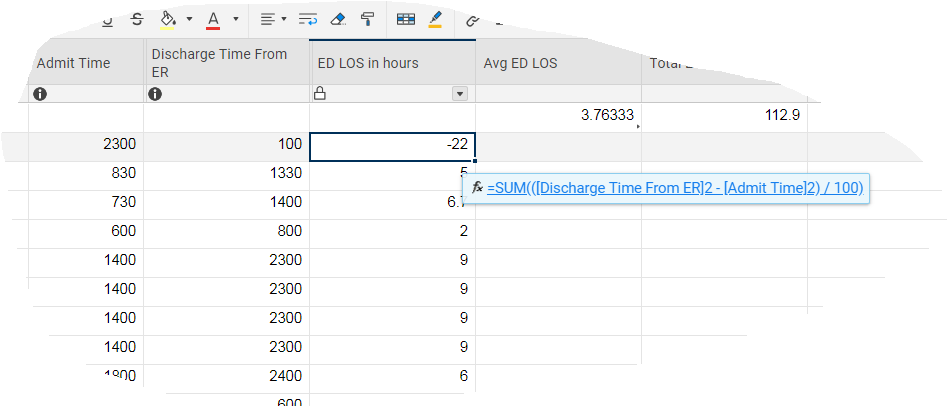# =SUM - Military Time conditional calculation Formula

Need help with calculation of military time:

I have two columns that I am using to track Admission time and Discharged time to determine and average length of stay.

I am using military time for each column and storing the difference in a third column for each row

=SUM(([Discharge Time From ER]2 - [Admit Time]2) / 100)

Exp DC time 300 – Ad time 100 = 2 hrs

However I was notified that we will be keeping track of admission times and discharge times that span past midnight (2400).

So I will have the Scenario where my admission time is 2300 hrs and my discharge time is 0200 hrs

Which with the above calculation will give me a negative number and hose up my averages

So can someone assist in providing a formula where if my calculation is negative to add 24 to the negative number otherwise if positive leave the value alone?

I am not that experienced with Smartsheet formulas and conditionals and could use the help.

I have provided a snapshot below.• sorry attached a picture of example• Basically, the formula you are requesting would be this:

=IF(SUM(([Discharge Time]@row - [Admit Time]@row) / 100) < 0, SUM(([Discharge Time]@row - [Admit Time]@row) / 100) + 24, SUM(([Discharge Time]@row - [Admit Time]@row) / 100))

This should calculate correctly as long as all the stays are less than 24 hours and in one hour increments, e.g. not 0830, 1215, etc.

• Thank you for your help, it works perfectly, I appreciate your skill and time!

## Help Article Resources

Want to practice working with formulas directly in Smartsheet?

Check out the Formula Handbook template!